# User:Tohline/SR/EOS

## Equations of State

### Ideal Gas

The ideal gas equation of state is referred to as the ideal gas law in Wikipedia. The Wikipedia discussion points out that, as derived from first principles in statistical mechanics, this "gas law" can most naturally be written in the form,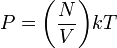$P = \biggl( \frac{N}{V} \biggr) kT$

where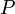$~P$,$~T$ and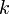$~k$ are as we have defined them in our accompanying variables appendix, and the ratio (N / V) specifies the number density of free particles that make up the gas. The number density of free particles also can be written as a ratio of the mass density,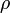$~\rho$, to the average mass per free particle,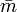$\bar{m}$, that is, the ideal gas equation of state may be written in the form,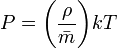$P = \biggl( \frac{\rho}{\bar{m}} \biggr) kT$ .

It is customary in astrophysical discussions to write the average mass per free particle as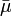$~\bar{\mu}$mu, that is, as a product of the atomic mass unit, mu = 1/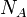$~N_A$, and a dimensionless coefficient of order unity referred to as the the mean molecular weight,$~\bar{\mu}$. This leads to what we will refer to as,

Form A
of the ideal gas equation of state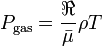$~P_\mathrm{gas} = \frac{\Re}{\bar{\mu}} \rho T$
,

where the gas constant,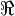$~\Re$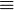$\equiv$$~k$$~N_A$.

 © 2014 - 2020 by Joel E. Tohline |   H_Book Home   |   YouTube   | Appendices: | Equations | Variables | References | Ramblings | Images | myphys.lsu | ADS |